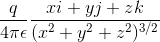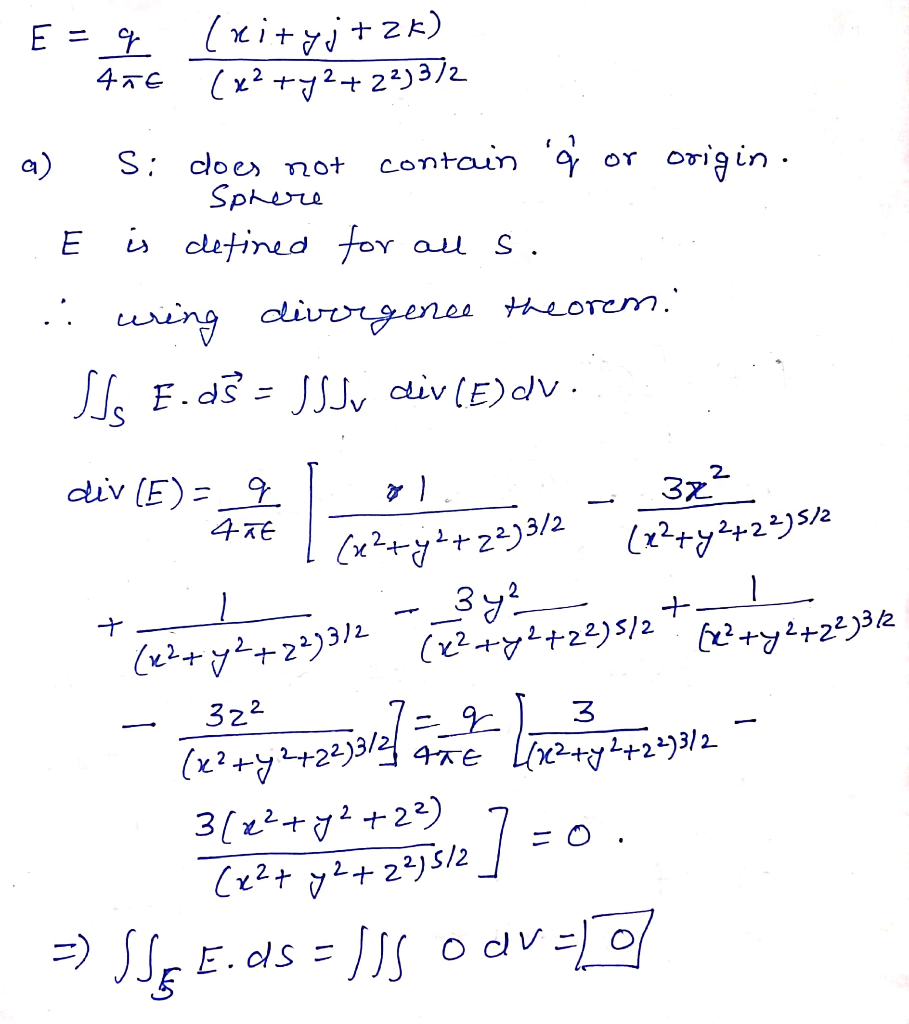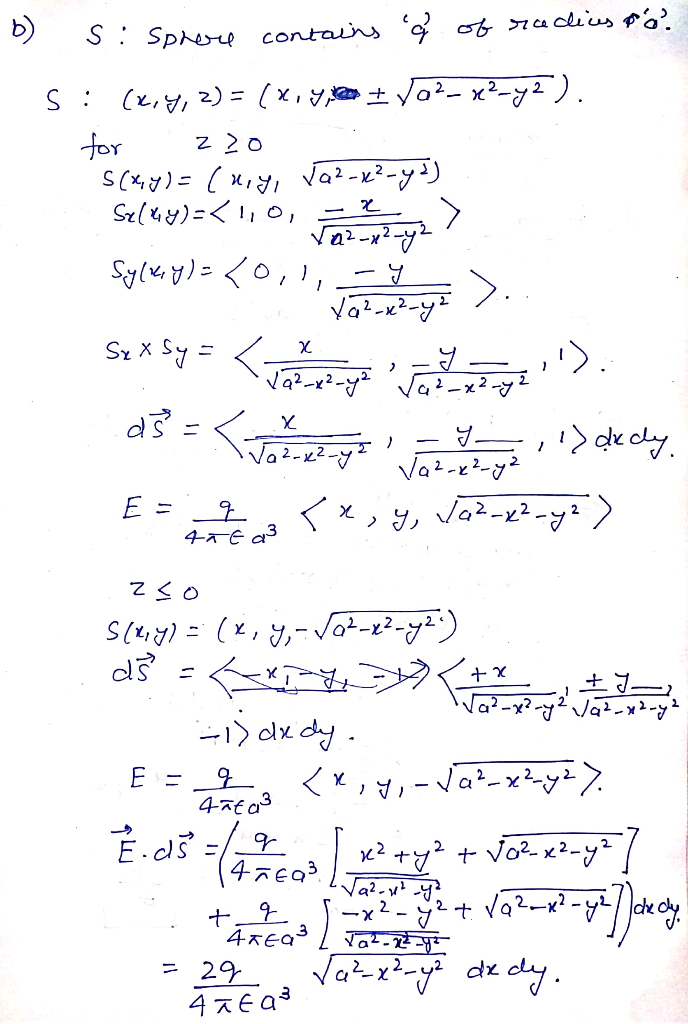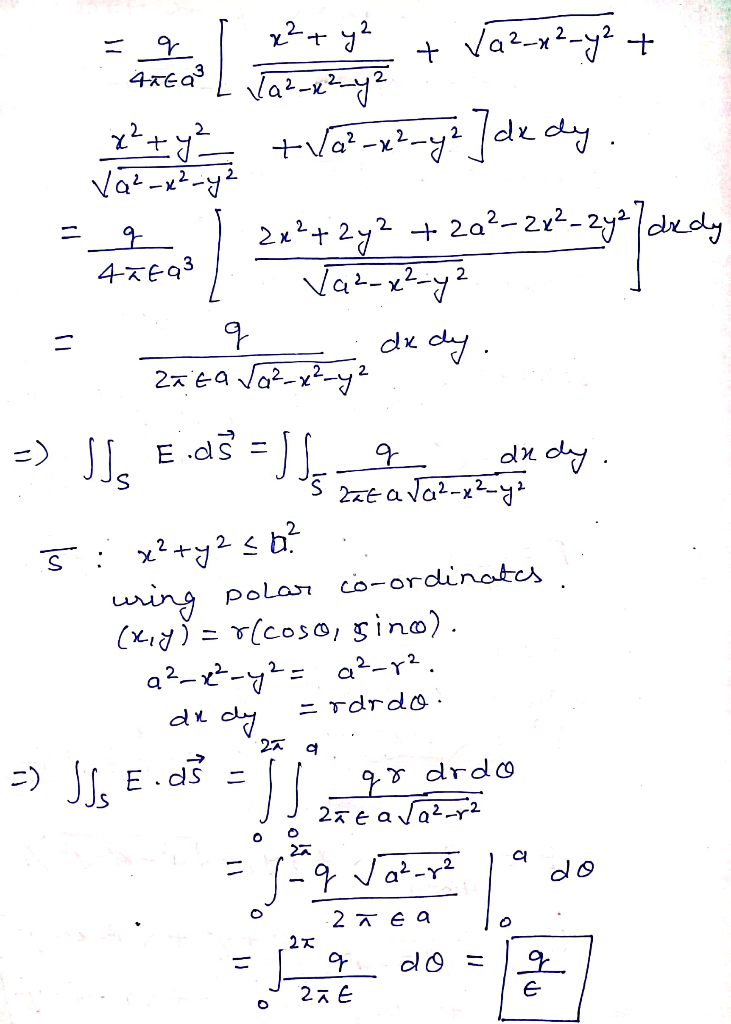# In electromagnetic theory, the electric eld created by a point charge q located at the origin is E = where is a const...

In electromagnetic theory, the electric eld created by a point charge q located at the origin is E =whereis a constant.

Let S be a sphere in R3.

(a) Find the outward flux of E across S, if S does not contain q.

(b) Find the outward flux of E across S, if S is centered at q.

iyjzk 4TE (r2y 2)3/2##### Add Answer of: In electromagnetic theory, the electric eld created by a point charge q located at the origin is E = where is a const...
More Homework Help Questions Additional questions in this topic.

• #### A point charge of -7 C is located at x = 3 m, y = -2 m. A second point charge of 12 C is located...

Need Online Homework Help?